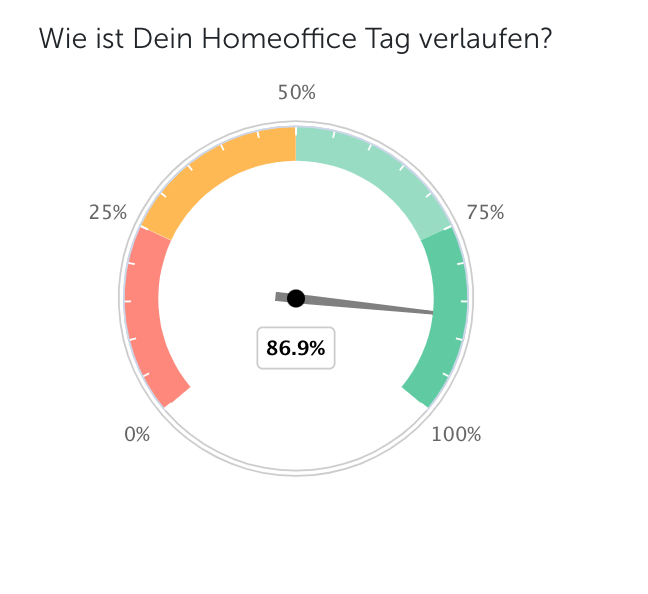# How are the Honestly Charts calculated?

#### Example ChartHow exactly is the result calculated?

For this example, we have chosen a Likert scale with 5 items.

The answer options are worth 0-4 points in descending order ("very good" = 4 points; "very poor" = 0 point).

The average (mean) of all answers is calculated. To do this, the frequency and score of the given answer are multiplied by each other, divided by the total number of answers, and then converted into a percentage value, where a value of 100% represents the maximum achievable result.

For this example, the result is calculated as follows:

135 feedbacks were collected:

#feedback = 135

Thereof:

80 times "very good" = 80*4 = 320

41 times "good" = 41*3 = 123

12 times "okay" = 12*2 = 24

2 times "bad" = 2*1 = 2

0 times "very bad" = 0*0 = 0

This results in a total score of:

320 + 123 + 24 + 2 + 0 = 469

The maximum total score achievable with 135 feedbacks:

135 * 4 = 540

The final result is calculated as follows:

469/540 ≈ 0,869

0,869* 100 = 86,9%

Accordingly, the rounded result displayed in the gauge chart is: 86.9%